Smartick is a fun way to learn math!Feb02

# Prime Numbers Activities with Smartick

In previous posts we’ve learned about prime numbers and composite numbers. Today we’re going to look at different sequenced prime numbers activities involving prime numbers that Smartick students work on. We’ve included examples of different types and levels, from identifying whether a number is prime or composite to finding the least common multiple and highest common factor through prime factor decomposition. Let’s jump right in with the first example:

Index

## Identifying Prime Numbers

This activity asks students to identify whether a number is prime or composite.

###7 is a prime number because it is only divisible by 1 and 7.

## Factorization of Composite Numbers

These types of exercises require the student to find three different multiplications that give the same result (the composite number).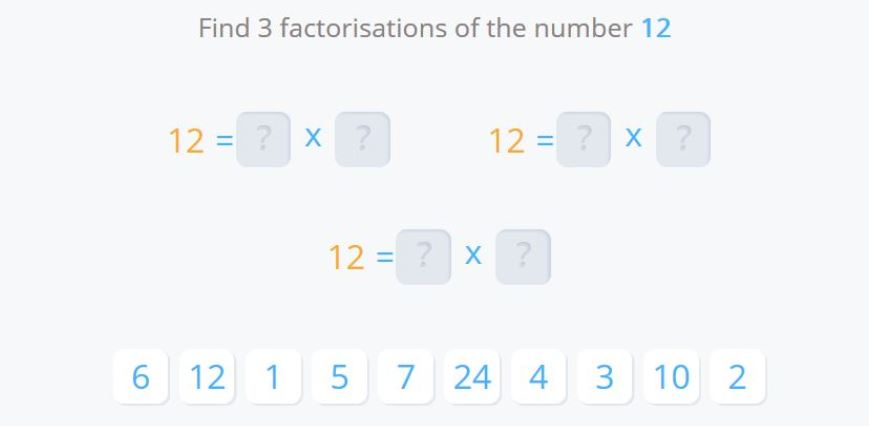In this example there are three solutions:

The first would be to multiply 1 x 12, the second 2 x 6 and the third 3 x 4. This shows us that the number is not prime, because it is divisible by other numbers other than 1 and itself. We also learn that there are other possibilities, in addition to factorization with prime numbers.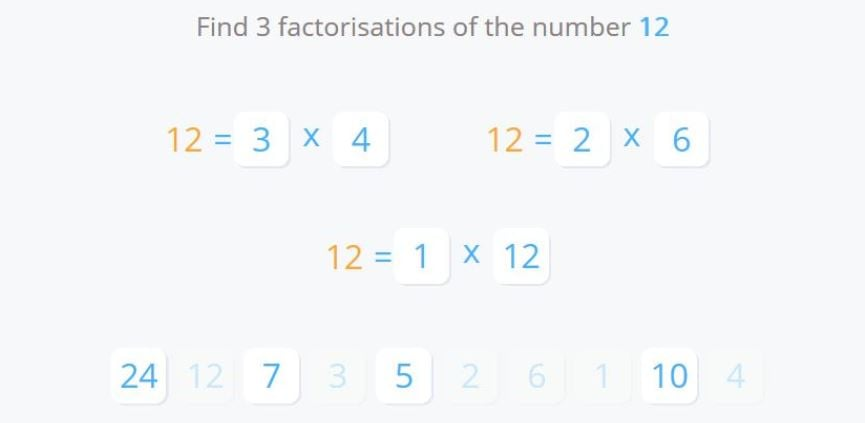## Prime Numbers and Rules of divisibility

We learned about divisibility criteria in a previous post. There are also Smartick exercises that require us to work out which numbers are prime and which aren’t, by applying these criteria.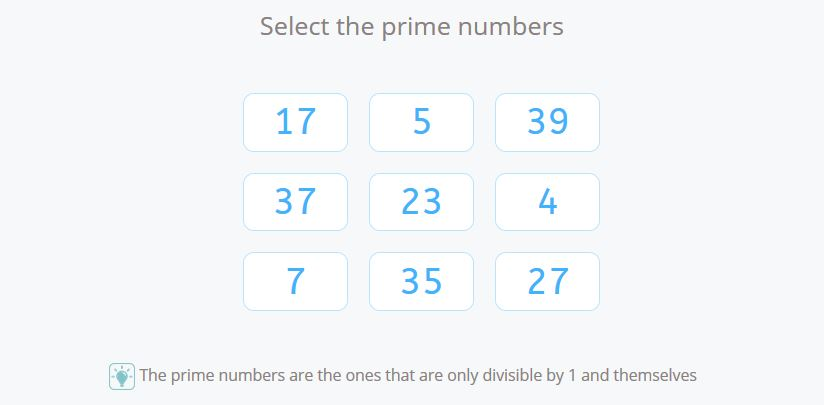We have to select the numbers 5, 7, 17, 23, and 37. These numbers are only divisible by 1 and themselves. The rest are divisible by other numbers. Following the divisibility criteria, 4 is divisible by 2, 27 is divisible by 3, 35 is divisible by 5 and 39 is divisible by 3. Therefore they are not prime numbers.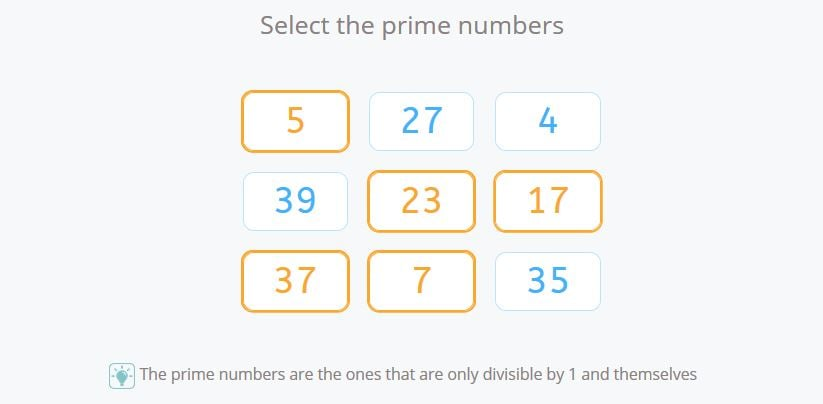## Finding the Highest Common Factor through Factorization with Prime Numbers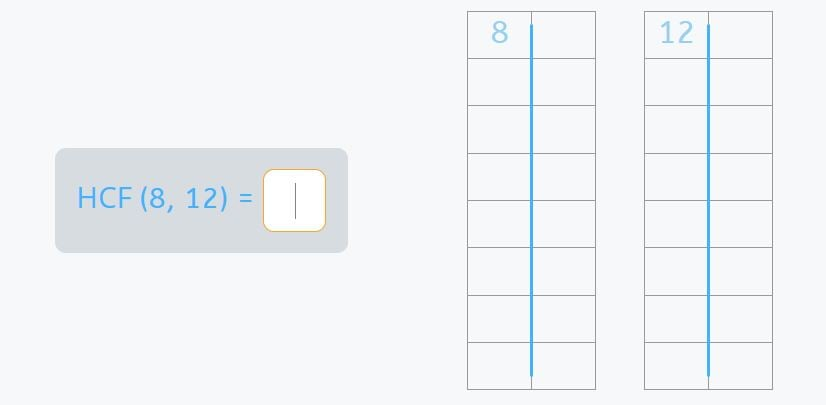To find the HCF of 8 and 12, we need to decompose these two numbers into their prime factors and find the common factors raised to the smallest exponent.

8 = 2 x 2 x 2, and 12 = 2 x 2 x 3. The only common factor is 2, and the smallest exponent it is elevated to is also 2. 2 x 2 = 4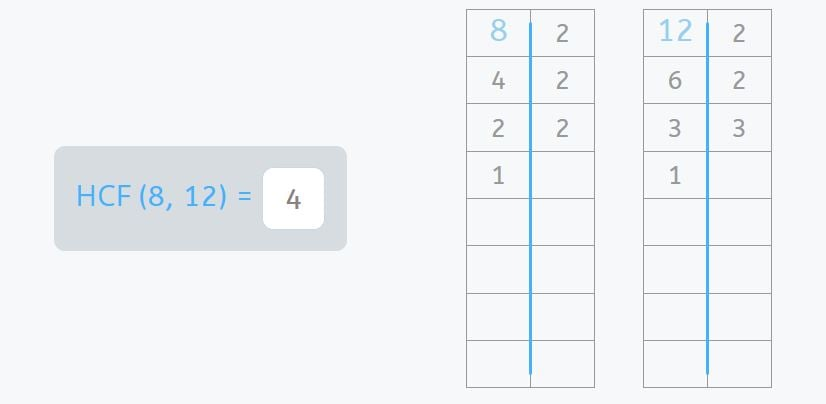If you want to keep practicing exercises involving prime numbers activities and much more, you can log in to Smartick and try it for free to learn much more elementary math!

Fun is our brain’s favorite way of learning
Diane Ackerman
Smartick is a fun way to learn math
• 15 fun minutes a day
• Millions of students since 2009